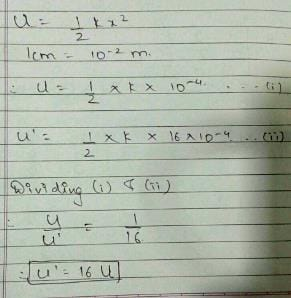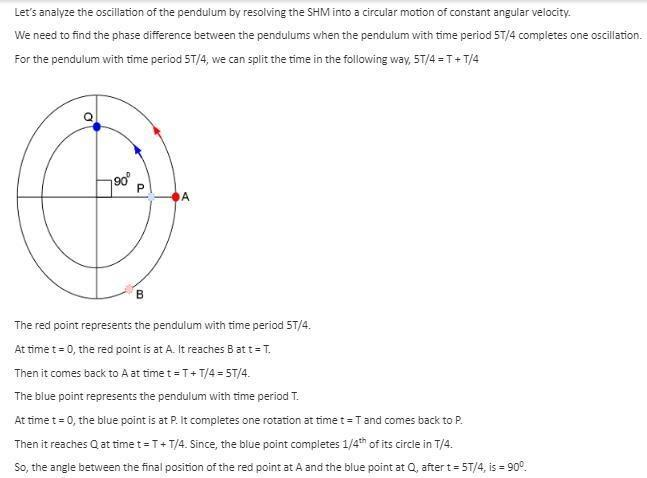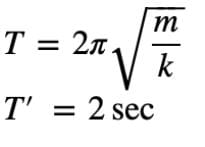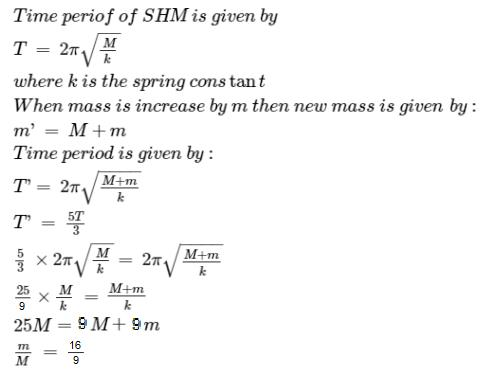NEET  >  Test: Oscillations

# Test: Oscillations

Test Description

## 30 Questions MCQ Test Physics Class 11 | Test: Oscillations

Test: Oscillations for NEET 2022 is part of Physics Class 11 preparation. The Test: Oscillations questions and answers have been prepared according to the NEET exam syllabus.The Test: Oscillations MCQs are made for NEET 2022 Exam. Find important definitions, questions, notes, meanings, examples, exercises, MCQs and online tests for Test: Oscillations below.
Solutions of Test: Oscillations questions in English are available as part of our Physics Class 11 for NEET & Test: Oscillations solutions in Hindi for Physics Class 11 course. Download more important topics, notes, lectures and mock test series for NEET Exam by signing up for free. Attempt Test: Oscillations | 30 questions in 30 minutes | Mock test for NEET preparation | Free important questions MCQ to study Physics Class 11 for NEET Exam | Download free PDF with solutions
 1 Crore+ students have signed up on EduRev. Have you?
Test: Oscillations - Question 1

### The pendulum of a wall clock executes

Detailed Solution for Test: Oscillations - Question 1

The bob of a pendulum moves in such a way that it repeats its positions several time but alternately the time gap between these positions is always equal which proves that the motion of a pendulum is oscillatory.

Test: Oscillations - Question 2

### In a simple pendulum the restoring force is due to

Detailed Solution for Test: Oscillations - Question 2

In a simple pendulum the restoring force is due to the tangential component of the gravitational force because on applying torque equation on the radial forces the effect is nullified due to it passing from centre & only tangential component remains.

Test: Oscillations - Question 3

### The kinetic energy of a body executing S.H.M. is 1/3 of the potential energy. Then, the displacement of the body is x percent of the amplitude, where x is

Detailed Solution for Test: Oscillations - Question 3

We know that PE + KE = TE = constant
Hence at the extremis TE = PE = ½ kz2
Where z is amplitude and k is shm constant.
Thus when KE = ⅓ PE
We get PE = ¾ TE =  ½ kz2
Hence we get  ½ kx2 = ¾  ½ kz2
We get x/z = √3/4
= 1.73 / 2
= .87
Thus we get x is 87 percent of the amplitude.

Test: Oscillations - Question 4

If the length of a simple pendulum is increases by 1percent, then the new time-period

Detailed Solution for Test: Oscillations - Question 4

Time period, T=2π√l/g
=>T∝√l
=>ΔT/T=1/2 Δl/l
Δl/l=1%
Therefore, ΔT/T=1/2x1%=0.5%

Test: Oscillations - Question 5

A body is executing S.H.M When its displacement from the mean position is 4 cm and 5 cm, the corresponding velocities of the body are 10 cm per sec and 8 cm per sec. Then the time period of the body is

Detailed Solution for Test: Oscillations - Question 5

v=ω√A2−x2
​⇒8=ω √A2−25​
&10=ω√A2−16
​⇒(8/10​)2= A2−25/ A2−16
​⇒16/25​= A2−25/ A2−16
​⇒16A2−256=25A2−625
⇒9A2=369
A2=41
⇒8=ω41−25
​⇒8=4ω
∴ω=2=T2π​
T=π sec​

Test: Oscillations - Question 6

Oscillatory motion is

Detailed Solution for Test: Oscillations - Question 6

Oscillatory motion is periodic to and fro motion. By definition

Test: Oscillations - Question 7

In a damped system

Test: Oscillations - Question 8

A spring with spring constant k when stretched through 1 cm has a potential energy U. If it is stretched by 4 cm, the potential energy will become

Detailed Solution for Test: Oscillations - Question 8Test: Oscillations - Question 9

Two pendulums have time periods T and 5T/4. They are in phase at their mean positions at some instant of time. What will be their phase difference when the bigger pendulum completes one oscillation?

Detailed Solution for Test: Oscillations - Question 9Test: Oscillations - Question 10

The ratio of maximum acceleration to maximum velocity of a particle performing S.H.M is equal to

Detailed Solution for Test: Oscillations - Question 10

Maximum acceleration = w2A
Maximum velocity = wA
Ratio = w2A/wA = w
w = angular velocity
A = amplitude

Test: Oscillations - Question 11

Periodic motion is

Test: Oscillations - Question 12

Damping is due to

Detailed Solution for Test: Oscillations - Question 12

Damping is due to resistive forces like air drag, friction etc.

Test: Oscillations - Question 13

Two pendulums of length 1 m and 16 m start vibrating one behind the other from the same stand. At some instant, the two are in the mean position in the same phase. The time period of shorter pendulum is T. The minimum time after which the two threads of the pendulum will be one behind the other is

Detailed Solution for Test: Oscillations - Question 13

T1=2π√1/g=T, T2=2π√16/g=4T
(ω1−ω2)t=2π
(2π/T−2π/4T)t=2π
t=4T/3

Test: Oscillations - Question 14

The period of a simple pendulum is doubled when

Test: Oscillations - Question 15

A particle is executing S.H.M with an amplitude of 4 cm and time period 12 sec. The time taken by the particle in going from its mean position to a position of displacement equal to 2 cm is T1..The time taken from this displaced position of 2 cm to reach the extreme position is T2. Therefore, T1/ T2 will be

Test: Oscillations - Question 16

Period of a periodic motion is the ________ interval of time after which the motion is __________.

Detailed Solution for Test: Oscillations - Question 16

Explanation:Time period of an oscillatory motion is the smallest interval of time for one complete oscillation.As when we apply the force on the bob,bob moves from mean position to extreme position then form extreme position to mean position and then again from mean to extreme in opposite direction and at last come to mean position.The time taken by bob in this whole process is called period of oscillation.

Test: Oscillations - Question 17

In simple models damping force is

Test: Oscillations - Question 18

For all practical purposes, the motion of a simple pendulum is SHM,

Detailed Solution for Test: Oscillations - Question 18

For all practical purposes, the motion of a simple pendulum is SHM only if the maximum angle which the string makes with the vertical is less than 1 because for angles less than 1° sinθ = θ.

Test: Oscillations - Question 19

Whose motion is an ideal case of SHM?

Detailed Solution for Test: Oscillations - Question 19

Simple pendulum is not a case of ideal SHM as it is mention in NCERT.
Shadow of a point mass having uniform circular motion on the horizontal diameter is a case of SHM but not on the vertical diameter.

Test: Oscillations - Question 20

If the period of oscillation of mass M suspended from a spring is one second, then the period of mass 4 M will be

Detailed Solution for Test: Oscillations - Question 20

T=2*3.14*√m/√k=1 s (given)

T’=2*3.14*√4m/√k=2*3.14*2√m/√k=2*1s

Therefore T’=2sTest: Oscillations - Question 21

Frequency of the periodic motion is

Test: Oscillations - Question 22

Damped natural frequency is

Detailed Solution for Test: Oscillations - Question 22

If a resonant mechanical structure is set in motion and left to its own devices, it will continue to oscillate at a particular frequency known as its natural frequency, or "damped natural frequency". This will be a little lower in frequency than the resonant frequency, which is the frequency it would assume if there were no damping. The resonant frequency is also called the "undamped natural frequency”

Test: Oscillations - Question 23

A rubber ball with water, having a small hole in its bottom is used as the bob of a simple pendulum. The time-period of such a pendulum:

Detailed Solution for Test: Oscillations - Question 23

When rubber ball completely filled with water its centre of gravity will be at its centre, as water will fall through hole its COG will shift towards lower side leading to increase in length of pendulum and thus T, when very small amount of water will be left in rubber ball its COG will again shifts upward causing decrease in length and thus T, and finally when rubber ball becomes empty its COG will be at its centre and T will remains same as earlier.

Test: Oscillations - Question 24

A particle executes S.H.M. having time period T, then the time period with which the potential energy changes is:

Test: Oscillations - Question 25

The length of the second’s pendulum on the surface of earth is 1m. The length of second’s pendulum on the surface of moon, where g is 1/6th the value on earth is

Detailed Solution for Test: Oscillations - Question 25

Time period remains same since
T = 2π √(L/g)
So, from proportionality we can solve

Test: Oscillations - Question 26

Any periodic function can be expressed as a.

Detailed Solution for Test: Oscillations - Question 26

All periodic functions can, in principle, be represented as a sum of sine and cosine functions, since they form what's known as a complete set.

Test: Oscillations - Question 27

In forced oscillations apart from acceleration forces, damping and spring forces there is

Test: Oscillations - Question 28

A mass M is suspended from a spring of negligible mass. The spring is pulled a little and then released so that the mass executes simple harmonic oscillations with a time period T. If the mass is increased by m, the time period becomes 5T/3. then the ratio of m/M is

Detailed Solution for Test: Oscillations - Question 28Test: Oscillations - Question 29

The length of a second’s pendulum decreases by 0.1percent, then the clock

Detailed Solution for Test: Oscillations - Question 29

L’=1.001L
T=2π√L/9=1s
T’=2π√L’/g=2π√1.001/g=Tx√1.001=1.0005xT
T’=1.0005s
Error in time per day=1.0005x60x60x24-1x60x60x24
Error=86443.2-86400
Error=43.2s

Test: Oscillations - Question 30

If the maximum velocity and acceleration of a particle executing S.H.M are equal in magnitude, the time period will be

## Physics Class 11

126 videos|449 docs|210 tests
 Use Code STAYHOME200 and get INR 200 additional OFF Use Coupon Code
Information about Test: Oscillations Page
In this test you can find the Exam questions for Test: Oscillations solved & explained in the simplest way possible. Besides giving Questions and answers for Test: Oscillations, EduRev gives you an ample number of Online tests for practice

## Physics Class 11

126 videos|449 docs|210 tests

### How to Prepare for NEET

Read our guide to prepare for NEET which is created by Toppers & the best Teachers